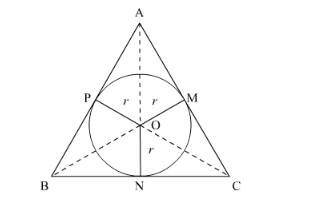# If area of a circle inscribed in an equilateral triangleQuestion:

If area of a circle inscribed in an equilateral triangle is 48π square units, then perimeter of the triangle is

(a) $17 \sqrt{3}$ units

(b) 36 units

(c) 72 units

(d) $48 \sqrt{3}$ units

Solution:

Let the circle of radius be inscribed in an equilateral triangle of side a.Area of the circle is given as 48π.

$\Rightarrow \pi r^{2}=48 \pi$

$\Rightarrow r^{2}=48$

$\Rightarrow r=4 \sqrt{3}$

Now, it is clear that ONBC. So, ON is the height of ΔOBC corresponding to BC.

Area of ΔABC = Area of ΔOBC + Area of ΔOCA + Area of ΔOAB = 3 × Area of ΔOBC

$\frac{\sqrt{3}}{4} \times a^{2}=3 \times \frac{1}{2} \times \mathrm{BC} \times \mathrm{ON}$

$\frac{\sqrt{3}}{4} \times a^{2}=3 \times \frac{1}{2} \times a \times r$

$\frac{\sqrt{3}}{4} \times a^{2}=3 \times \frac{1}{2} \times a \times 4 \sqrt{3}$

$a=24$

Thus, perimeter of the equilateral triangle = 3 × 24 units = 72 units